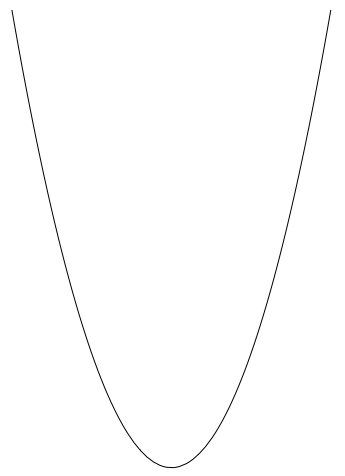### Home > ACC7 > Chapter 9 Unit 10 > Lesson CC3: 9.2.1 > Problem9-60

9-60.Cisco was looking at a table of values from a silent board game of $y = x^{2}$. She said, “This table contains $(0, 0)$, so I think it shows a proportional relationship.” Is Cisco correct? Why or why not?

Proportional relationships do contain the point $(0, 0)$, but is she sure that the graph makes a straight line or that the table has points with corresponding ratios?

Examine the graph of $x^{2}$ to the right.
Why is Cisco incorrect?